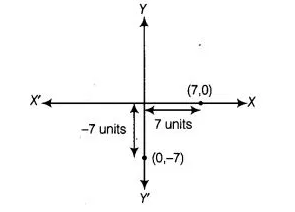# A point lies on positive direction of X-axis at`
Question:

A point lies on positive direction of X-axis at a distance of 7 units from the Y-axis. What are its coordinates? What will be the coordinates, if it lies on

negative direction of Y-axis at a distance of 7 units from X-axis?

Solution:

Given, point lies on the positive direction of X-axis, so its y-coordinate will be zero and it is at a distance of 7 units from the X-axis, so its coordinates

are (7, 0). If it lies on negative direction of X-axis, then its x-coordinate will be zero and its distance from X-axis is 7 units, so its coordinates are (0, -7).